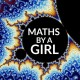# Galton Board

Standard

Like previous post, in this post I will discuss another contribution of Jacob (Jacques) Bernoulli. The motivation for this post came from Cédric Villain’s recent TED talk. Though I am not a fan of probability theory, but this “toy”, which I am going to discuss, is really interesting.  Consider following illustration from a journal’s cover:Source: https://goo.gl/3kWnK5

“Galton Board” was invented by Francis Galton in 1894.  It provided a remarkable way to visualize the distribution obtained by performing several Bernoulli Trials in pre-digital computer era.  Bernoulli trial is the simplest possible random experiment with exactly two possible outcomes, “success” and “failure”, in which the probability of success (say, p) is the same every time the experiment is conducted.  If we perform these Bernoulli trials more than one time (say, n times) we get, what we call, Binomial Distribution. We get a discrete distribution like this:And  when the number of Bernoulli trials is very large (theoretically what we would call infinite number of trials), this Binomial Distribution can be approximated to Normal Distribution, which is a continuous distribution.Source: http://goo.gl/aQGv7V

The Normal Distribution is important because of the Central Limit Theorem. This theorem implies that if you have many independent variables that may be generated by all kinds of distributions, assuming that nothing too crazy happens, the aggregate of those variables will tend toward a normal distribution. This universality across different domains of science makes the normal distribution one of the centerpieces of applied mathematics and statistics.

Here is a video in which James Grime demonstrates how Galton Board can be used to visualize Normal Distribution approximation of Binomial Distribution for very large number of Bernoulli trials. The trial outcome are represented graphically as a path in the Galton board: success corresponds to a bounce to the right and failure to a bounce to the left.## About gaurish

Life is never fair. So I made my life a mathematical fair.

### 5 responses

1.mathsbyagirl on said:

Funny, I just finished my last statistics exam where I had to study this! Note that the Binomial distribution can only be approximated to the normal distribution if p is similar to 0.5. 🙂

Like

•gaurish on said:

p need not be similar to 0.5; normal distribution curve can also be a skewed one, what you are claiming is true for “standard normal distribution” which we use to make Z-score table. see: https://surfstat.anu.edu.au/surfstat-home/3-2-8.html

Like

•mathsbyagirl on said:

Ah! Thanks for the clarification 🙂

Liked by 1 person